### IMO Shortlist 1988 problem 15

Kvaliteta:
Avg: 0,0
Težina:
Avg: 0,0
Let$ABC$ be an acute-angled triangle. The lines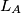$L_{A}$,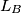$L_{B}$ and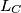$L_{C}$ are constructed through the vertices$A$,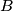$B$ and$C$ respectively according the following prescription: Let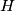$H$ be the foot of the altitude drawn from the vertex$A$ to the side$BC$; let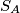$S_{A}$ be the circle with diameter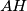$AH$; let$S_{A}$ meet the sides$AB$ and$AC$ at$M$ and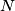$N$ respectively, where$M$ and$N$ are distinct from$A$; then let$L_{A}$ be the line through$A$ perpendicular to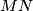$MN$. The lines$L_{B}$ and$L_{C}$ are constructed similarly. Prove that the lines$L_{A}$,$L_{B}$ and$L_{C}$ are concurrent.
Izvor: Međunarodna matematička olimpijada, shortlist 1988